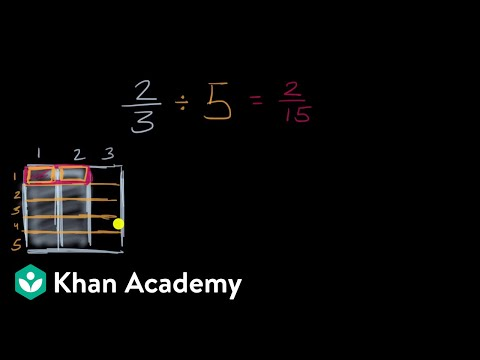# Understanding division of fractions (Full video)

Description: Using a number line, we'll explain why multiplying by the inverse is the same as dividing. Created by Sal Khan. Let's think about what it means to take 8/3 and divide it by 1/3. So let me draw a number line here.

### Other videos you might be interested in### Dividing Fractions Word Problems (Full video)

#### Student Achievement Partners